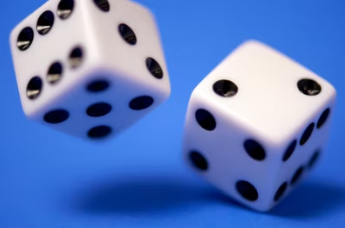## Dicey Probability

You throw two 6-sided dice. What is the probability that the sum will be greater than 2?Hint
There is only one throw (1 and 1) that sums to 2 (snake eyes).
Hint 2
There are a total of 36 possible rolls for two dice (6 x 6 = 36).
There is only one throw (1 and 1) that sums to 2 (snake eyes). There are also 36 possible rolls for two dice (6 x 6 = 36). Therefore, 35/36 will have a sum greater than 2.
$$\frac{35}{36}=0.9721\:probability$$$$$0.9721\:probability$$$